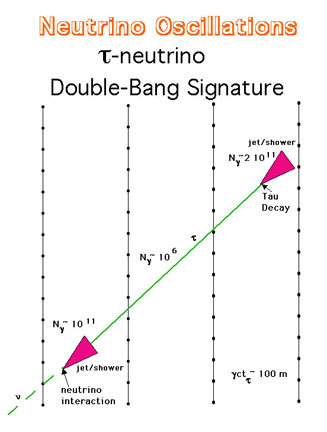## Detecting Tau-Neutrino by KM3

The KM3 detector can be used to detect PeV energy tau-neutrinos.

## Introduction

The tau-neutrino has never been detected. Its existence is only inferred by missing energy and momentum and analogy to the electron and muon each of which have their corresponding neutrino and anti-neutrino to conserve their lepton number and energy & momentum.

## Scientific Motivation

Ultra-high-energy tau-neutrinos are likely to be produced in small numbers, roughly the 10^{-4} level of the muon and electron neutrino numbers. In thermal production, e.g. supernova at few MeV, GRBs at 100 MeV, one would expect that all flavors of neutrinos would be produced in equal numbers, similar to the equilibrium that existed early in the Big Bang. However, at high energies, specifically PeV energies, one expects that the pion (decaying to muons and muon neutrinos with the muons decaying to electrons plus muon and electron neutrinos) production is the most copius channel for neutrino production. Tau-neutrinos are likely to arise from Charm meson and direct tau-lepton production which are much suppressed compared to pion production.

Thus unless the neutrino detector is extremely large, observing a tau-nuetrino is relatively unlikely and rare. Howeer, if there are neutrino oscillations, then a significant number of tau-neutrinos can result. (This assumes a reasonable, at least finite, mixing angle.) Then one has the very real possibility to detect PeV energy tau-neutrinos by their "doubl"e-bang signature.

more to come this is a place holder

## Concept

Because of time dilation, the lifetime of a PeV tau leads to a mean decay distance of order 100 m. If a PeV energy tau-neutrino interacts in the detector, it will produce a first "bang" of energy deposition when a nucleon is struck and a tau-meson is produced. Then a minimum ionizing particle emerges from the first cascade and travels about 100 meters, to decay into another mini-jet of energy deposition.

This figure shows the geometry of such an event and the amount of light (number of photons in the OM bandwidth) expected from each of the three components of the double-bang signature.Reference to Papers relating to detecting PeV tau-neutrinos:

Title: Detecting Tau-Neutrino Oscillations at PeV Energies
Authors: John G. Learned and Sandip Pakvasa (University of Hawaii)
(hep-ph 9405296)

xxxx

xxxx

## KM3 Project

KM3 a telescope designed to detect and identify point sources of high-energy (greater than 1 TeV) neutrinos